## Helmholtz Differential Equation--Parabolic Cylindrical Coordinates

In Parabolic Cylindrical Coordinates, the Scale Factors are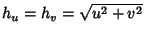,and the separation functions are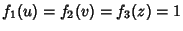, giving Stäckel Determinant of. The Helmholtz Differential Equation is(1)

Attempt Separation of Variables by writing(2)

then the Helmholtz Differential Equation becomes(3)

Divide by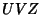,(4)

Separating thepart,(5)(6)(7)

so(8)

which has solution(9)

and(10)

This can be separated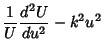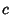(11)(12)

so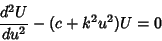(13)(14)

These are the Weber Differential Equations, and the solutions are known as Parabolic Cylinder Functions.